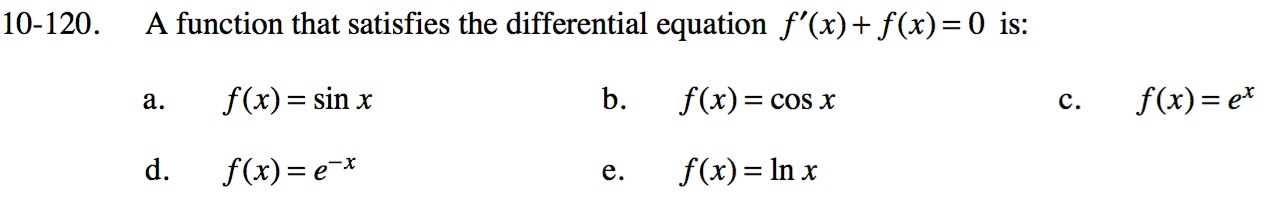### Home > CALC > Chapter 10 > Lesson 10.3.1 > Problem10-120

10-120.
1. A function that satisfies the differential equation f ′(x) + f(x) = 0 is: Homework Help ✎

1. f(x) = sin x

2. f(x) = cos x

3. f(x) = ex

4. f(x) = ex

5. f(x) = ln x$\text{Integrate: }\ln|y|=-x+C$

$y=Ce^{-x}$